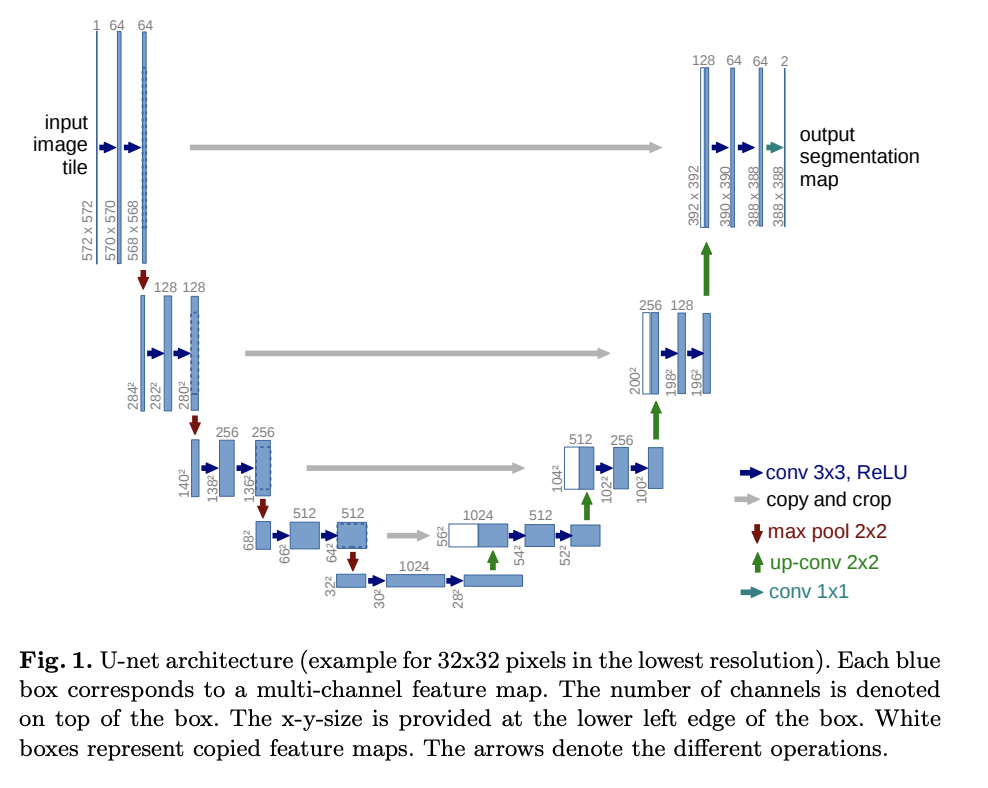# U-Net

This is an implementation of the U-Net model from the paper, U-Net: Convolutional Networks for Biomedical Image Segmentation.

U-Net consists of a contracting path and an expansive path. The contracting path is a series of convolutional layers and pooling layers, where the resolution of the feature map gets progressively reduced. Expansive path is a series of up-sampling layers and convolutional layers where the resolution of the feature map gets progressively increased.

At every step in the expansive path the corresponding feature map from the contracting path concatenated with the current feature map.Here is the training code for an experiment that trains a U-Net on Carvana dataset.

27import torch
28import torchvision.transforms.functional
29from torch import nn

### Two Convolution Layers

Each step in the contraction path and expansive path have two convolutional layers followed by ReLU activations.

In the U-Net paper they used padding, but we use padding so that final feature map is not cropped.

32class DoubleConvolution(nn.Module):
• in_channels is the number of input channels
• out_channels is the number of output channels
43    def __init__(self, in_channels: int, out_channels: int):
48        super().__init__()

First convolutional layer

51        self.first = nn.Conv2d(in_channels, out_channels, kernel_size=3, padding=1)
52        self.act1 = nn.ReLU()

Second convolutional layer

54        self.second = nn.Conv2d(out_channels, out_channels, kernel_size=3, padding=1)
55        self.act2 = nn.ReLU()
57    def forward(self, x: torch.Tensor):

Apply the two convolution layers and activations

59        x = self.first(x)
60        x = self.act1(x)
61        x = self.second(x)
62        return self.act2(x)

### Down-sample

Each step in the contracting path down-samples the feature map with a max pooling layer.

65class DownSample(nn.Module):
73    def __init__(self):
74        super().__init__()

Max pooling layer

76        self.pool = nn.MaxPool2d(2)
78    def forward(self, x: torch.Tensor):
79        return self.pool(x)

### Up-sample

Each step in the expansive path up-samples the feature map with a up-convolution.

82class UpSample(nn.Module):
89    def __init__(self, in_channels: int, out_channels: int):
90        super().__init__()

Up-convolution

93        self.up = nn.ConvTranspose2d(in_channels, out_channels, kernel_size=2, stride=2)
95    def forward(self, x: torch.Tensor):
96        return self.up(x)

### Crop and Concatenate the feature map

At every step in the expansive path the corresponding feature map from the contracting path concatenated with the current feature map.

99class CropAndConcat(nn.Module):
• x current feature map in the expansive path
• contracting_x corresponding feature map from the contracting path
106    def forward(self, x: torch.Tensor, contracting_x: torch.Tensor):

Crop the feature map from the contracting path to the size of the current feature map

113        contracting_x = torchvision.transforms.functional.center_crop(contracting_x, [x.shape, x.shape])

Concatenate the feature maps

115        x = torch.cat([x, contracting_x], dim=1)
117        return x

## U-Net

120class UNet(nn.Module):
• in_channels number of channels in the input image
• out_channels number of channels in the result feature map
124    def __init__(self, in_channels: int, out_channels: int):
129        super().__init__()

Double convolution layers for the contracting path. The number of features gets doubled at each step starting from .

133        self.down_conv = nn.ModuleList([DoubleConvolution(i, o) for i, o in
134                                        [(in_channels, 64), (64, 128), (128, 256), (256, 512)]])

Down sampling layers for the contracting path

136        self.down_sample = nn.ModuleList([DownSample() for _ in range(4)])

The two convolution layers at the lowest resolution (the bottom of the U).

139        self.middle_conv = DoubleConvolution(512, 1024)

Up sampling layers for the expansive path. The number of features is halved with up-sampling.

143        self.up_sample = nn.ModuleList([UpSample(i, o) for i, o in
144                                        [(1024, 512), (512, 256), (256, 128), (128, 64)]])

Double convolution layers for the expansive path. Their input is the concatenation of the current feature map and the feature map from the contracting path. Therefore, the number of input features is double the number of features from up-sampling.

149        self.up_conv = nn.ModuleList([DoubleConvolution(i, o) for i, o in
150                                      [(1024, 512), (512, 256), (256, 128), (128, 64)]])

Crop and concatenate layers for the expansive path.

152        self.concat = nn.ModuleList([CropAndConcat() for _ in range(4)])

Final convolution layer to produce the output

154        self.final_conv = nn.Conv2d(64, out_channels, kernel_size=1)
• x input image
156    def forward(self, x: torch.Tensor):

To collect the outputs of contracting path for later concatenation with the expansive path.

161        pass_through = []

Contracting path

163        for i in range(len(self.down_conv)):

Two convolutional layers

165            x = self.down_conv[i](x)

Collect the output

167            pass_through.append(x)

Down-sample

169            x = self.down_sample[i](x)

Two convolutional layers at the bottom of the U-Net

172        x = self.middle_conv(x)

Expansive path

175        for i in range(len(self.up_conv)):

Up-sample

177            x = self.up_sample[i](x)

Concatenate the output of the contracting path

179            x = self.concat[i](x, pass_through.pop())

Two convolutional layers

181            x = self.up_conv[i](x)

Final convolution layer

184        x = self.final_conv(x)
187        return x